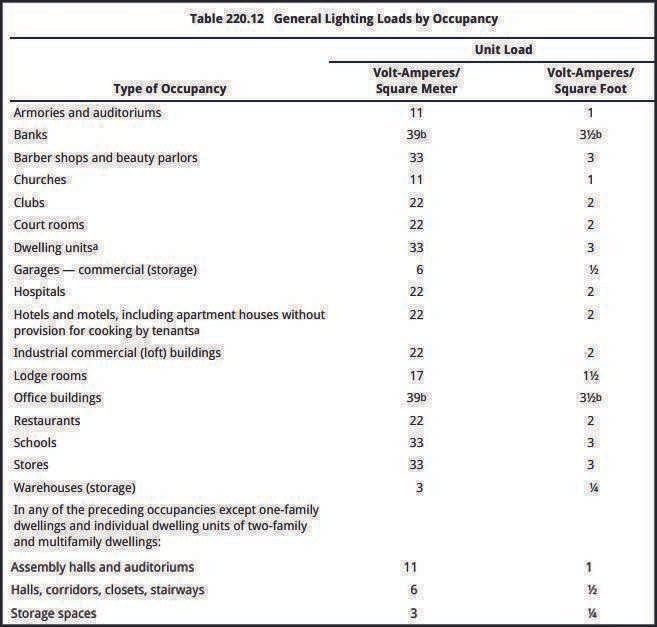# What is Demand Factor and Diversity Factor? Quick Concepts

## What is Demand Factor and Diversity Factor?Lighting Load Demand Factors Based on Occupancy – Compliant with NEC Article 220 – Understanding electrical demand factors for lighting loads according to occupancy as per the National Electrical Code (NEC) Article 220.

Subscribe to our Newsletter “Electrical Insights Daily” to get the latest updates in Electrical Engineering. You can also Follow us LinkedIn to see our latest posts.

• ### What is the demand factor?

The demand factor refers to the ratio of the maximum demand of a system to the total connected load.

• ### What is the formula for the demand factor?

The formula for the demand factor is: Demand Factor = Maximum Demand / Total Connected Load.

• ### What is demand factor and its importance?

The demand factor represents the relationship between the maximum demand and the total connected load, indicating the extent to which the actual demand deviates from the total load.

• ### What is the diversity factor and demand factor?

The diversity factor and demand factor are both measures used in electrical engineering. The diversity factor relates to the ratio of the sum of individual maximum demands to the maximum demand of the whole system, while the demand factor specifically focuses on the ratio of maximum demand to the total connected load.

• ### Why is diversity factor used?

The diversity factor is used to account for the fact that not all loads connected to a system operate simultaneously at their maximum levels, allowing for more accurate sizing of electrical equipment and optimization of system design.

• ### Can demand factor be more than 1?

No, the demand factor cannot be more than 1. It represents the ratio of maximum demand to the total connected load, so it is always less than or equal to 1.

• ### What is the demand factor as per IEC standard?

The demand factor as per the International Electrotechnical Commission (IEC) standard is not specified as a fixed value. The IEC provides guidelines and standards for electrical systems but does not dictate a specific demand factor value.

• ### How is the demand factor calculated?

The demand factor is calculated by dividing the maximum demand of a system or equipment by the total connected load. The formula is:
Demand Factor = Maximum Demand / Connected Load

• ### How is the diversity factor calculated?

The diversity factor is calculated by dividing the sum of individual maximum demands by the maximum demand of the group. The formula is:
Diversity Factor = Sum of Individual Maximum Demands / Maximum Demand of Group

• ### What is the difference between demand factor and diversity factor?

The demand factor relates to the ratio of the actual maximum demand to the total connected load, while the diversity factor relates to the ratio of the sum of individual maximum demands to the maximum demand of a group.

• ### Can demand factor and diversity factor be greater than 1?

Yes, both demand factor and diversity factor can be greater than 1. This occurs when the maximum demand or sum of individual maximum demands exceeds the connected load or maximum demand of the group, respectively.

Sharing is Caring